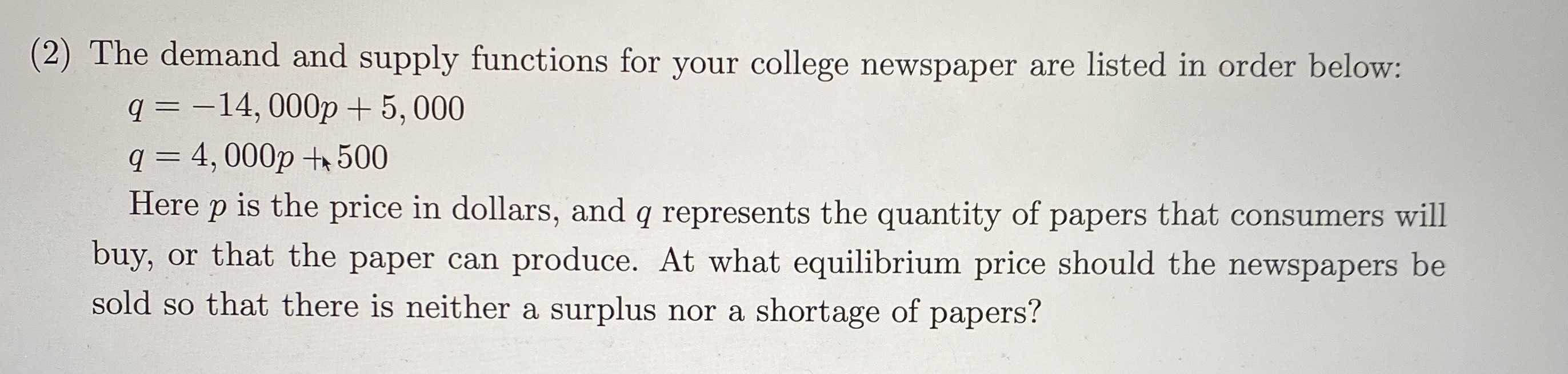### ¿Todavía tienes preguntas de matemáticas?

Pregunte a nuestros tutores expertos
Algebra
Pregunta(2) The demand and supply functions for your college newspaper are listed in order below:

$$q = - 14,000 p + 5,000$$ $$q = 4,000 p + 500$$

Here p is the price in dollars, and $$q$$ represents the quantity of papers that consumers will buy, or that the paper can produce. At what equilibrium price should the newspapers be sold so that there is neither a surplus nor a shortage of papers?